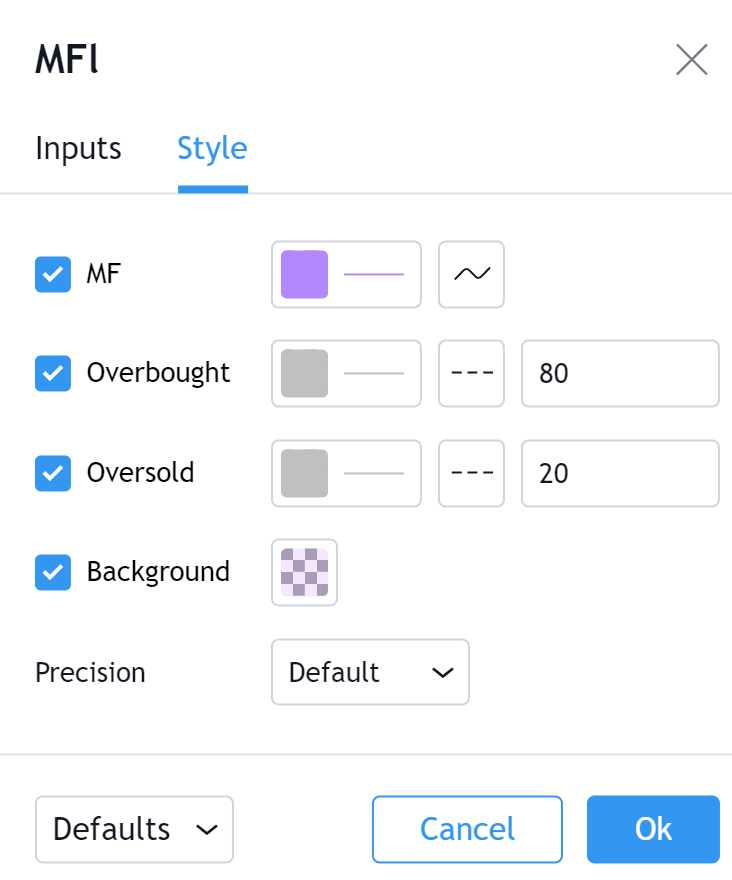# Money Flow (MFI)

#### Definition

The Money Flow Index indicator (MFI) is a tool used in technical analysis for measuring buying and selling pressure. This is done through analyzing both price and volume. The MFI's calculation generates a value that is then plotted as a line that moves within a range of 0-100, making it an oscillator. When the MFI rises, this indicates an increase in buying pressure. When it falls, this indicates an increase in selling pressure. The Money Flow Index can generate several signals, most notably: overbought and oversold conditions, divergences, and failure swings.

#### History

The Money Flow Index indicator (MFI) was created by Gene Quong and Avrum Soudack.

#### Calculation

There are four separate steps to calculate the Money Flow Index. The following example is for a 14 Period MFI:

1. Calculate the Typical Price

`(High + Low + Close) / 3 = Typical Price`

2. Calculate the Raw Money Flow

`Typical Price x Volume = Raw Money Flow`

3. Calculate the Money Flow Ratio

`(14 Period Positive Money Flow) / (14 Period Negative Money Flow)`

Positive Money Flow is calculated by summing the Money Flow of all of the days in the period where Typical Price is higher than the previous period Typical Price.

Negative Money Flow is calculated by summing the Money Flow of all of the days in the period where Typical Price is lower than the previous period Typical Price.
4. Calculate the Money Flow Index.

`100 - 100/(1 + Money Flow Ratio) = Money Flow Index)`

#### The basics

The Money Flow Index (MFI) is actually quite similar to The Relative Strength Index (RSI). The RSI is a leading indicator used to measure momentum. The MFI is essentially the RSI with the added aspect of volume. Because of its close similarity to RSI, the MFI can be used in a very similar way.

#### What to look for

##### Overbought/Oversold

When momentum and price rise fast enough, at a high enough level, eventual the security will be considered overbought. The opposite is also true. When price and momentum fall far enough they can be considered oversold. Traditional overbought territory starts above 80 and oversold territory starts below 20. These values are subjective however, and a technical analyst can set whichever thresholds they choose.

##### Divergence

MFI Divergence occurs when there is a difference between what the price action is indicating and what MFI is indicating. These differences can be interpreted as an impending reversal. Specifically there are two types of divergences, bearish and bullish.
Bullish MFI Divergence – When price makes a new low but MFI makes a higher low.

Bearish MFI Divergence – When price makes a new high but MFI makes a lower high.

##### Failure Swings

Failure swings are another occurrence which can lead to a price reversal. One thing to keep in mind about failure swings is that they are completely independent of price and rely solely on MFI. Failure swings consist of four steps and are considered to be either Bullish (buying opportunity) or Bearish (selling opportunity).

###### Bullish MFI Failure Swing
1. MFI drops below 20 (considered oversold).
2. MFI bounces back above 20.
3. MFI pulls back but remains above 20 (remains above oversold)
4. MFI breaks out above its previous high.
###### Bearish MFI Failure Swing
1. MFI rises above 80 (considered overbought)
2. MFI drops back below 80
3. MFI rises slightly but remains below 80 (remains below overbought)
4. MFI drops lower than its previous low.

#### Summary

A financial instrument's price and its correlation with momentum is a very important metric for any technical analyst. Because of this, the Money Flow Index (MFI) can be a very valuable technical analysis tool. Of course, MFI should not be used alone as the sole source for a traders signals or setups. MFI can be combined with additional indicators or chart pattern analysis to increase its effectiveness.

#### Inputs##### Length

The time period to be used in calculating the MFI. 14 is the default.

#### Style##### MFI

Can toggle the visibility of the MFI as well as the visibility of a price line showing the actual current value of the MFI. Can also select the MFI Line's color, line thickness and visual style.

##### Precision

Sets the number of decimal places to be left on the indicator's value before rounding up. The higher this number, the more decimal points will be on the indicator's value.

Профиль Настройка профиля Счёт и оплата Ваши друзья Монеты Мои запросы в поддержку Справочный центр Личные сообщения Чат Выйти